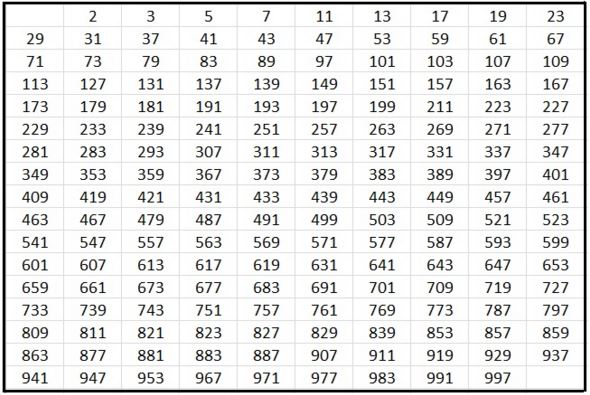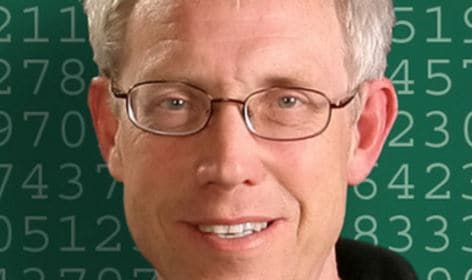The largest prime number ever, with 22,338,618 digits, has been discovered by a computer at the University of Central Missouri in the United States, as part of the Great Internet Mersenne Prime Search (GIMPS), an international quest to find a particular type of large prime numbers.

A Prime Number is one that can be divided evenly only by itself or 1 – it must be greater than 1. For example, the number 5 can only be divided by 5 or 1, therefore it is a prime number. Six, however, can be divided by 1 and 6, and also by 2 and 3, so it is not a prime number – it is a composite number.

This new record-long prime number is five million digits longer than the previous record, which had also been found by the same computer.A prime number can only be divided by 1 and itself. Here is a list of some of them – there is an infinite number of prime numbers. (Image: www.whatareprimenumbers)

Number of possible prime numbers is infinite

You might think that there is one largest prime number, with no greater prime number possible. Although they become less frequent as the numbers get bigger, there are an infinite number of prime numbers.

Mathematicians say a prime number this big may prove useful in future computing.

The Great Internet Mersenne Prime Search (GIMPS), a collaborative project of volunteers who use freely available software to search for Mersenne prime numbers, celebrated its 20th birthday on January 7th “with the math discovery of the new largest known prime number – 274,207,281-1.”

The number was discovered by a computer volunteered by Dr. Curtis Cooper, Professor of Computer Science at the University of Central Missouri’s Department of Mathematics and Computer Science.

According to the Mersenne Research, Inc., the new giant prime number, also known as M74207281, is calculated by multiplying together 74,207,281 twos, and then subtracting one.Dr. Curtis Cooper’s computer discovered the new prime number.

49th known Mersenne prime

It is the 49th known Mersenne prime ever discovered – they are becoming increasingly difficult to find.

A Mersenne prime is a prime number that is one less than a power of two, i.e. it is a prime number that can be written in the form Mn=2n – 1, for some integer n. They are named after Marin Mersenne (1588-1648), a French theologian, philosopher, mathematician and music theorist, often referred to as the ‘father of acoustics’, who studied them in the 17th century.

In the GIMPS project, volunteers download a free program to search for these giant Mersenne primes. There is a cash prize for whoever computes the biggest prime.

It took 1 month to check the calculation

It took 31 days to check that Dr. Cooper’s computer had done the calculation correctly.

“The primality proof took 31 days of non-stop computing on a PC with an Intel I7-4790 CPU. To prove there were no errors in the prime discovery process, the new prime was independently verified using both different software and hardware.”

Dr. Cooper will receive a \$3,000 dollar prize. This is the fourth time he and his university have won the prize (2005, 2006 and 2013).

Dr. Cooper’s computer reported the new record-breaking prime in GIMPS on 17th September, 2015. However, nobody noticed it until routine maintenance data-mined it.